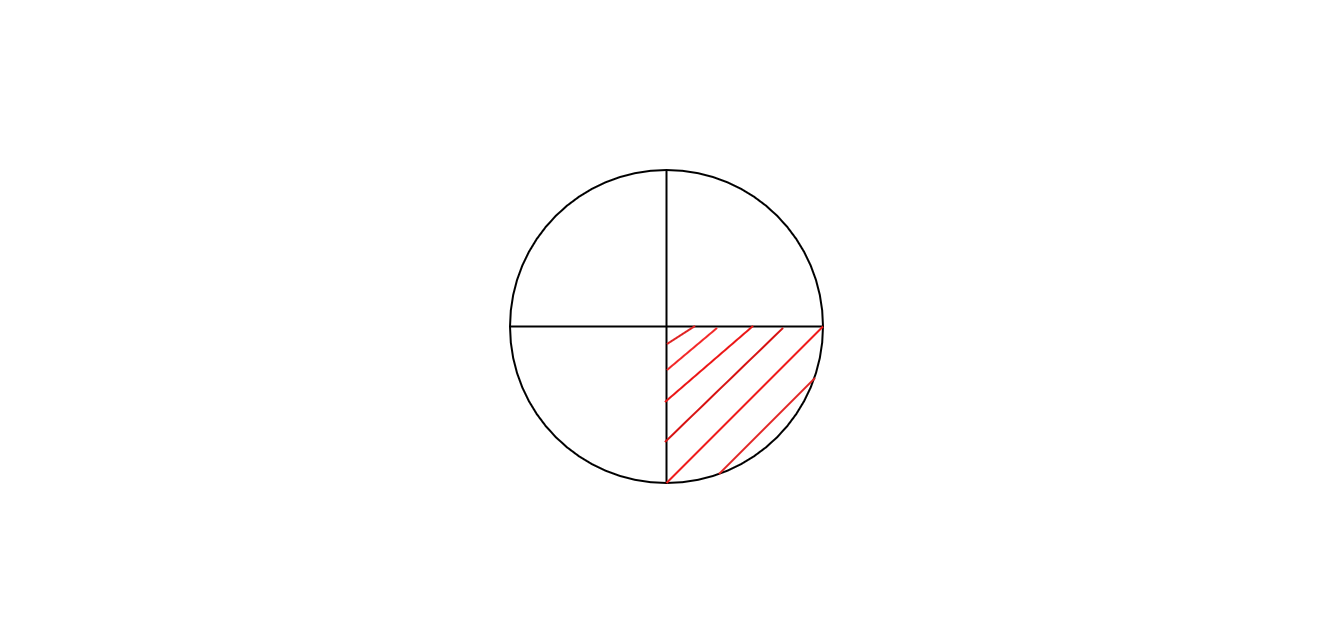"
">

# What part of the figure is shaded and hence find the % of the shaded part :"

Given :

The circle with four quadrants is given.

To find :

We have to find what part of the circle is shaded, and  % of the shaded part.

Solution :

Number of parts in the given figure = 4

Number of parts shaded = 1

The percentage of the shaded part $= \frac{Number \ of \ parts \ shaded}{Total \ number \ of \ parts} \times 100$

$=\frac{1}{4} \times 100$

= 25%.

1 part of figure is shaded and which is 25% of the given figure.

Updated on: 10-Oct-2022

23 Views